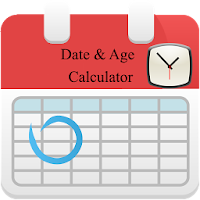Age Calculator -Age Calculator And Date Calculator# Age Calculator -Age Calculator And Date Calculator

Developer: Drifting Zone
App Size: 2.7M
Release Date: Sep 10, 2016
Price: Free
4.8
22 Ratings
Size
2.7M

### Screenshots for App

Mobile
This Age Calculator - Age Calculator And Date Calculator is a totally free app.
Now its really easy to calculate your age in days using this simple Age Calculator - Age Calculator And Date Calculator free app. In this Age Calculator - Age Calculator And Date Calculator you can calculate days left to any event or ceremony or you can calculate your service days , age limits days left , age in days or difference between two dates.
This Age Calculator - Age Calculator And Date Calculator free app will pick current date automatically which is change able (editable) which is totally for minimizing users headache of current date. You have to enter two dates and then click on calculate to get the time between between them in years months and days. For example you want to calculate your age then simply enter your date of birth in 2nd Date and in first date it will automatically pick current date and will show you , your age in Years , months and days.It totally free app and small size app.In every education or service field , it will help you a lot in timing saving. No calculation required to calculate your service tenure or age limitation, because this Age Calculator - Age Calculator And Date Calculator will do it in a second.In mostly education related fields they limit age for further education or scholarship, so this Age Calculator - Age Calculator And Date Calculator free app will calculate it with in no time. this age calculator is checked and verified.
This age calculator is tested by calculating many different ages and dates mathematically.